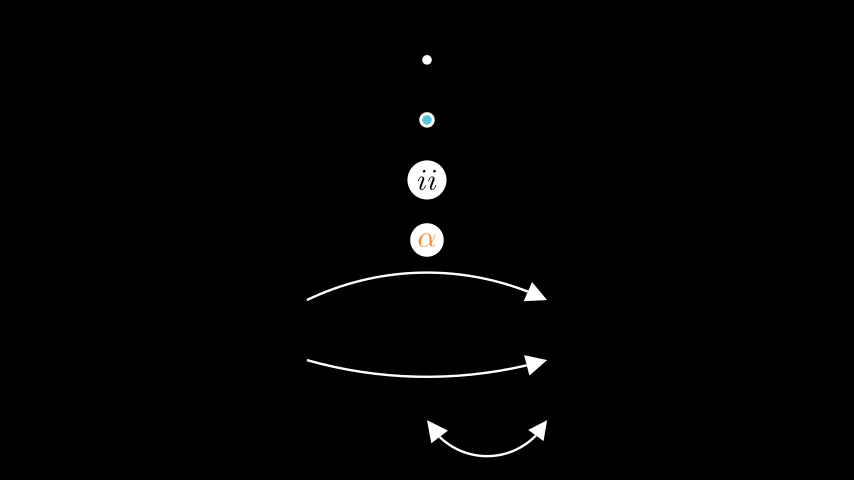# manim.mobject.geometry¶

Mobjects that are simple geometric shapes.

Examples##### UsefulAnnotations¶
class UsefulAnnotations(Scene):
def construct(self):
m0 = Dot()
m1 = AnnotationDot()
m2 = LabeledDot("ii")
m3 = LabeledDot(MathTex(r"\alpha").set_color(ORANGE))
m4 = CurvedArrow(2*LEFT, 2*RIGHT, radius= -5)
m5 = CurvedArrow(2*LEFT, 2*RIGHT, radius= 8)
m6 = CurvedDoubleArrow(ORIGIN, 2*RIGHT)

self.add(m0, m1, m2, m3, m4, m5, m6)
for i, mobj in enumerate(self.mobjects):
mobj.shift(DOWN * (i-3))


Classes

 Angle A circular arc or elbow-type mobject representing an angle of two lines. AnnotationDot A dot with bigger radius and bold stroke to annotate scenes. AnnularSector Annulus Arc A circular arc. ArcBetweenPoints Inherits from Arc and additionally takes 2 points between which the arc is spanned. ArcPolygon A generalized polygon allowing for points to be connected with arcs. ArcPolygonFromArcs A generalized polygon allowing for points to be connected with arcs. Arrow An arrow. ArrowCircleFilledTip Circular arrow tip with filled tip. ArrowCircleTip Circular arrow tip. ArrowSquareFilledTip Square arrow tip with filled tip. ArrowSquareTip Square arrow tip. ArrowTip Base class for arrow tips. ArrowTriangleFilledTip Triangular arrow tip with filled tip. ArrowTriangleTip Triangular arrow tip. Circle A circle. CubicBezier Example CurvedArrow CurvedDoubleArrow Cutout A shape with smaller cutouts. DashedLine A dashed Line. Dot A circle with a very small radius. DoubleArrow An arrow with tips on both ends. Elbow Two lines that create a right angle about each other: L-shape. Ellipse A circular shape; oval, circle. LabeledDot A Dot containing a label in its center. Line Polygon A shape created by defining its vertices. Rectangle A quadrilateral with two sets of parallel sides. RegularPolygon An n-sided regular polygon. RightAngle An elbow-type mobject representing a right angle between two lines. RoundedRectangle A rectangle with rounded corners. Sector SmallDot Deprecated - A dot with small radius Square A rectangle with equal side lengths. TangentLine Constructs a line tangent to a VMobject at a specific point. TipableVMobject Meant for shared functionality between Arc and Line. Triangle An equilateral triangle. Vector A vector specialized for use in graphs.